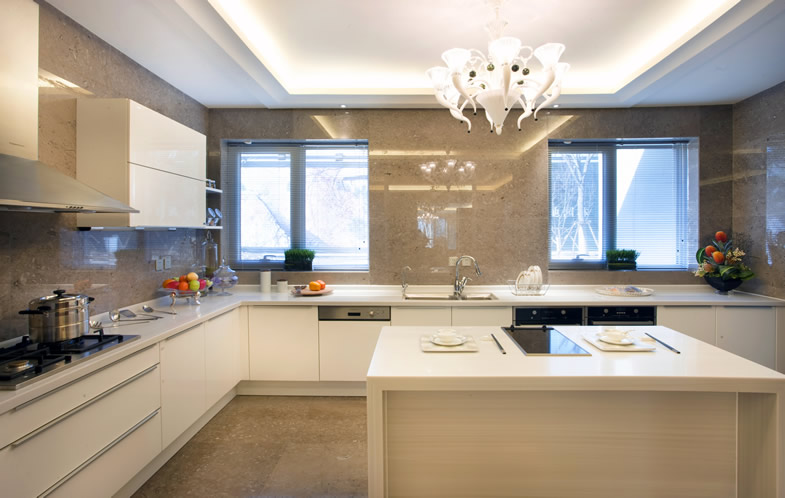# 整体厨房生意好做吗 整体厨房加盟费是多少

INTRODUCTION• 整体厨房是指在厨房内，将橱柜、厨具、厨电进行合理布局，达到整体配置、设计、施工装修的目的。相比于传统厨房，整体厨房能够有效地节省空间，功能性更强。随着我国整体厨房行业技术的不断发展以及人们生活消费水平的提高，整体厨房逐渐走进千家万户，并形成庞大的市场规模。在现代生活中，人们越来越重视生活质量，使得厨房逐渐成为人们享受生活的场景。目前我国整体厨房的覆盖率相对于发达国家较低，在未来行业市场增长空间巨大。

 加盟店型 省会城市 地级城市 县级城市 加盟店面积 120平米 120平米 120平米 装修费 8.4万元（700元/㎡） 7.2万元（600元/㎡） 6万元（500元/㎡） 经营设备费 9万元 8.5万元 8万元 首批进货费 7万元 6万元 5万元 宣传推广费用 6000元 5000元 4000元 店面租金 1.2万元/月 1万元/月 0.8万元/月 店员工资 12000元（4000元/人*3） 10800元（3600元/人*3） 9600元（3200元/人*3） 水电杂费 1200元 1100元 1000元 流动备用资金 10万元 8万元 6万元 加盟投资总费用 27.52万元 24.39万元 21.26万元

0*您的称呼
*代理区域
*手机号码
*意向行业
• 集成灶具
• 顶墙整装
• 地板材料
• 墙布墙纸
• 管道水管
• 净水器设备
• 全屋定制家居
• 艺术漆
• 油漆涂料
• 板材木材
• 门窗木门
• 智能锁门锁
• 学习桌椅
• 定制橱柜
• 瓷砖陶瓷
• 卫浴洁具
• 暖气片供暖
• 胶水美缝剂
• 古典红木家具
• 集成灶
• 集成水槽
• 燃气灶
• 油烟机
• 消毒柜
• 蒸箱
• 洗碗机
• 烤箱
• 集成吊顶
• 集成墙面
• 铝扣板
• 轻钢龙骨
• 浴霸
• 全屋整装
• 铝单板
• 地板
• 强化地板
• 实木地板
• 多层地板
• 竹地板
• 防静电地板
• 地热地板
• 塑胶地板
• 橡胶地板
• 软木地板
• 木塑地板
• 网络地板
• 弹性地板
• 运动地板
• 石塑地板
• 墙布
• 墙纸
• 水管管道
• 进口水管
• 穿线管
• 地暖管
• pp管
• ppr管
• pe管
• pvc管
• 净水器
• 纯水机
• 直饮机
• 饮水机
• 软水机
• 净水龙头
• 中央净水器
• 全屋定制
• 整体衣柜
• 整木家装
• 壁柜
• 衣柜门
• 榻榻米
• 玄关柜
• 板式家具
• 硅藻泥
• 艺术漆/艺术涂料
• 贝壳粉
• 腻子粉
• 油漆
• 乳胶漆
• 水性漆
• 木器漆
• 防火涂料
• 防水涂料
• 防腐涂料
• 建筑涂料
• 工业油漆
• 真石漆
• 粉末涂料
• 仿石涂料
• 氟碳漆
• 板材
• 生态板
• 细木工板
• 胶合板
• 饰面板
• 密度板
• 刨花板
• 集成材
• 防腐木
• 防火板
• 阻燃板
• 护墙板
• 吸音板
• 阳光板
• 装饰纸
• 人造板
• 碳化木
• 实木门
• 推拉门
• 防盗门
• 木门
• 室内门
• 免漆门
• 钢木门
• 防火门
• 玻璃门
• 静音门
• 生态门
• 时尚门
• 非标门
• 木塑门
• 楼宇门
• 铜门
• 折叠门
• 自动门
• 全屋门窗
• 铝合金门窗
• 不锈钢门窗
• 铝包木门窗
• 塑钢门窗
• 系统门窗
• 卷帘门窗
• 防盗窗
• 纱窗
• 隔音窗
• 防火窗
• 家装建材
• 门锁
• 智能锁/指纹锁
• 密码锁
• 防盗门锁芯
• 执手锁
• 酒店锁
• 挂锁
• 玻璃门锁
• 学习桌
• 书桌
• 电脑桌
• 桌子
• 儿童椅
• 课桌椅
• 电脑椅
• 办公桌
• 橱柜
• 不锈钢橱柜
• 整体厨房
• 瓷砖/地板砖
• 内墙砖
• 外墙砖
• 抛光砖
• 釉面砖
• 大理石瓷砖
• 木纹砖
• 玻化砖
• 马赛克瓷砖
• 通体砖
• 亚光砖
• 微晶石
• 仿古砖
• 大理石
• 石材石料
• 日用陶瓷
• 洁具卫浴
• 花洒
• 水龙头
• 马桶
• 智能马桶
• 台盆
• 浴室柜
• 浴缸
• 按摩浴缸
• 淋浴房
• 蹲便器
• 感应洁具
• 地漏
• 整体卫浴
• 小便斗
• 角阀
• 卫浴五金
• 全卫定制
• 水槽
• 冲水箱
• 马桶盖
• 暖气片
• 采暖器
• 供暖
• 美缝剂
• 瓷砖胶
• 石材胶
• 防水胶
• 结构胶
• 发泡胶
• 界面剂
• 玻璃胶
• 腻子
• 红木家具
• 古典家具
• 实木家具
• 榆木家具
• 分体式集成灶
• 高端建材
• 外国建材
• 家居建材
• 灯具建材
• 新型建材
• 五金建材
• 建材市场
*您的留言